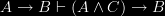# Question

What is a suitable name for the third property here?

1. Monotonicity:$A \rightarrow B \vdash (A \wedge C) \rightarrow B$
2. Conjunction:$(A \rightarrow B) \wedge (A \rightarrow C) \vdash A \rightarrow (B \wedge C)$
3. ???:$(A \rightarrow C) \wedge (B \rightarrow D) \vdash (A \wedge B) \rightarrow (C \wedge D)$

## 2 thoughts on “Question”

1.Pietro Galliani says:

Personally, I’d call 2. Right-conjunction, or R-Conjunction for brief; and then call 3. Right-Left-Conjunction, or RL-Conjunction. Then L-Conjunction would be the (rather trivial, at least in classical logic)

(A -> C) & (B -> C) |- (A & B -> C).

It might cause confusion with the right and left rules of sequent calculus, though…

2.admin says:

Wow, a response. Thanks!